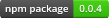infinity
Infinite sequences for Node.js. Supports typical array functions and more, all evaluated lazily.Last updated 7 years ago by rprieto .
Repository · Bugs · Original npm · Tarball · package.json
`\$ cnpm install infinity `
SYNC missed versions from official npm registry.

# infinity.js

Infinite sequences for Node.js

Supports typical array functions and more, all evaluated lazily.Joint effort of Andrew Jones, Maxim Filimonov, Leonardo Borges and Romain Prieto, during a ThoughtWorks Node.js hack night.

# Generators

``````infinite = require 'infinity'

infinite.range 9                        # naturals from 9 to infinity
infinite.cycle [1, 2, 3]                # repeats 1, 2, 3 forever
infinite.iteration 2, (i) -> i*2        # all powers of 2
infinite.zip s1, s2, s3                 # a steam pairing all given streams 1 to 1
infinite.cons 99, 98, stream            # a new stream starting with the given fixed values
infinite.fromArray [3, 6, 8]            # this one is actually finite, but still lazy
infinite.primes()                       # sequence of prime numbers
infinite.fibonacci()                    # fibonacci sequence
``````

# Operations

These operations create a new lazy stream:

``````stream = infinite.range 1

stream.filter (i) -> i%2 == 0       # a new stream with even numbers only
stream.map (i) -> i*2               # a new stream with all elements doubled
stream.skip 2                       # a new stream that starts 2 elements further
stream.takeWhile (i) -> i < 5       # a finite stream that stops at the given condition
``````

And these ones resolve a stream (non lazy):

``````# get the first 5 elements as a javascript array
stream.take 5

# reduce the stream to a single value
# can only be called on a finite stream (after takeWhile)
sum = (acc, i) -> acc + i
stream.reduce 0, sum
``````

# Example

FizzBuzz that doesn't use loops or modulo:

``````naturals = infinite.range 1
threes   = infinite.cycle ['', '', ' fizz']
fives    = infinite.cycle ['', '', '', '', ' buzz']
concat   = (arr) -> arr.join ''
infinite.zip(naturals, threes, fives).map(concat).take(20)
``````

# Custom streams

The basic generators (range, cycle) are not always enough.... but chances are you can express your stream as a head and a tail.

• The head is a function that returns a value
• The tail is a function that returns a stream

For example the following sequence outputs every second item it's given, lazily:

``````skipper = (s) -> infinite.stream (-> s.first()), (-> skipper s.tail().skip(1))

skipper(infinite.fibonacci()).take 5
# [ 0, 1, 3, 8, 21 ]
``````

This can be used to implement interesting algorithms. For example, here's the implementation of `infinite.primes()`:

``````primes = ->
sieve = (s) ->
infinite.stream (-> h), (-> sieve s.tail().filter (x) -> x % h isnt 0)
sieve(infinite.range 2)

infinite.primes().take 5
# [ 2, 3, 5, 7, 11 ]

infinite.primes().filter( (i) -> i > 1000 ).take 5
# [ 1009, 1013, 1019, 1021, 1031 ]
``````

Go to the folder you just cloned:

``````npm install
npm test
``````

## Current Tags

• 0.0.4                                ...           latest (7 years ago)

## 3 Versions

• 0.0.4                                ...           7 years ago
• 0.0.3                                ...           7 years ago
• 0.0.2                                ...           8 years ago